Processing ......FreeComputerBooks.com Links to Free Computer, Mathematics, Technical Books all over the World

Introduction to Probability for Data Science
Top Free Data Science Books 🌠 - 100% Free or Open Source!
• Title: Introduction to Probability for Data Science
• Author(s) Stanley H. Chan
• Publisher: Michigan Publishing Services (November 5, 2021)
• Hardcover/Paperback: 704 pages
• eBook: HTML and PDF
• Language: English
• ISBN-10/ASIN: 1607857464
• ISBN-13: 978-1607857464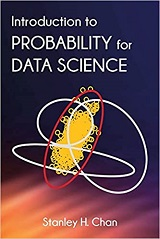Book Description

This book is an introductory textbook in undergraduate probability. It has a mission to spell out the motivation, intuition, and implication of the probabilistic tools we use in science and engineering. The writer has distilled what he believes to be the core of probabilistic methods. The author put the book in the context of data science to emphasize the inseparability between data (computing) and probability (theory) in our time.

It puts the probability in context with excellent examples from everyday science applications. The book presents examples in both Matlab and Python.

The book is a very good introduction for probability theory. In addition, it provides insightful explanations on those previous topics required for machine learning courses.

Reviews, Rating, and Recommendations: Related Book Categories: Read and Download Links:Similar Books:
•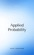Applied Probability (Paul Pfeiffer)

This book presents a unique blend of theory and applications, with special emphasis on mathematical modeling, computational techniques, and examples from the real world application in industries and science.

•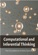Computational and Inferential: The Foundations of Data Science

Step by step, you'll learn how to leverage algorithmic thinking and the power of code, gain intuition about the power and limitations of current machine learning methods, and effectively apply them to real business problems.

•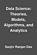Data Science: Theories, Models, Algorithms, and Analytics

It provides a bucket full of information regarding Data Science, covers a wide variety of sections by giving access to theories, data science algorithms, tools and analytics. You'll explore the right approach to best practices to guide you along the way.

•Python Data Science Handbook: Essential Tools (Jake VanderPlas)

Several resources exist for individual pieces of this data science stack, but only with the Python Data Science Handbook do you get them all - IPython, NumPy, Pandas, Matplotlib, Scikit-Learn, and other related tools.

•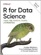R for Data Science: Visualize, Model, Transform, Tidy, Import

This book teaches you how to do data science with R: You'll learn how to get your data into R, get it into the most useful structure, transform it, visualize it and model it, how data science can help you work with the uncertainty and capture the opportunities.

•Introduction to Probability, Statistics, and Random Processes

This book introduces students to probability, statistics, and stochastic processes. It provides a clear and intuitive approach to these topics while maintaining mathematical accuracy. The book contains a large number of solved exercises.

•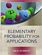Elementary Probability for Applications (Rick Durrett)

This clear and lively introduction to probability theory concentrates on the results that are the most useful for applications, including combinatorial probability and Markov chains. Concise and focused, it is designed for students familiar with basic calculus.

•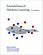Foundations of Machine Learning (Mehryar Mohri, et al)

This book is a general introduction to machine learning. It covers fundamental modern topics in machine learning while providing the theoretical basis and conceptual tools needed for the discussion and justification of algorithms.

Book Categories
 :All CategoriesTop Free BooksRecent BooksMiscellaneous BooksComputer EngineeringComputer LanguagesComputer ScienceData Science/DatabasesJava and Java EE (J2EE)Linux and UnixMathematicsMicrosoft and .NETMobile ComputingNetworking and CommunicationsSoftware EngineeringSpecial TopicsWeb Programming
Other Categories